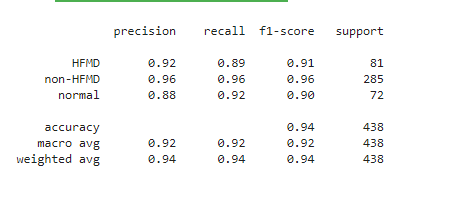# Precision, recall and f1-score for multiclass classification

I am trying out a multiclass classification setting with 7 classes. So how can I calculate the precision, recall, and f1-score for each class separately as follow? thanks.fastai has metrics for multiclass problems (see https://docs.fast.ai/metrics.html#Multi-label-classification).

You can use a one-against-all approach to calculate the respective scores. So if you have classes A, B, and C, the F1-scores would be calculated as A vs. non-A, B vs. non-B, and C vs. non-C.

Thank you so much.